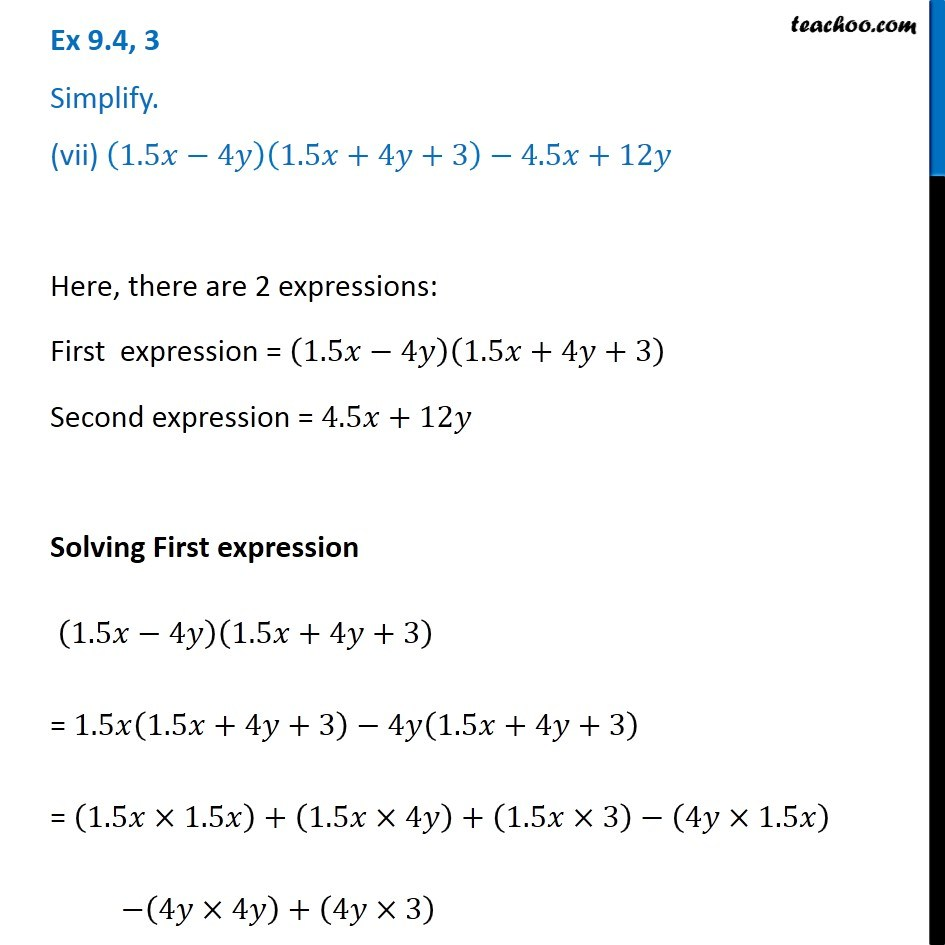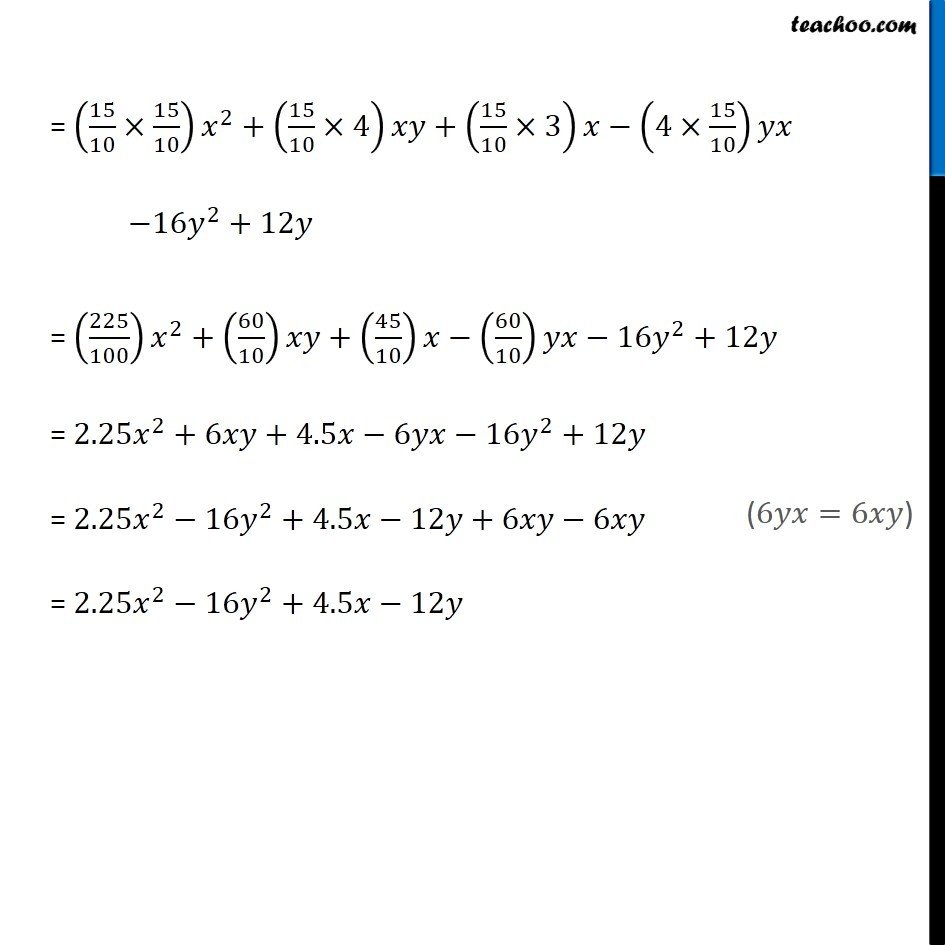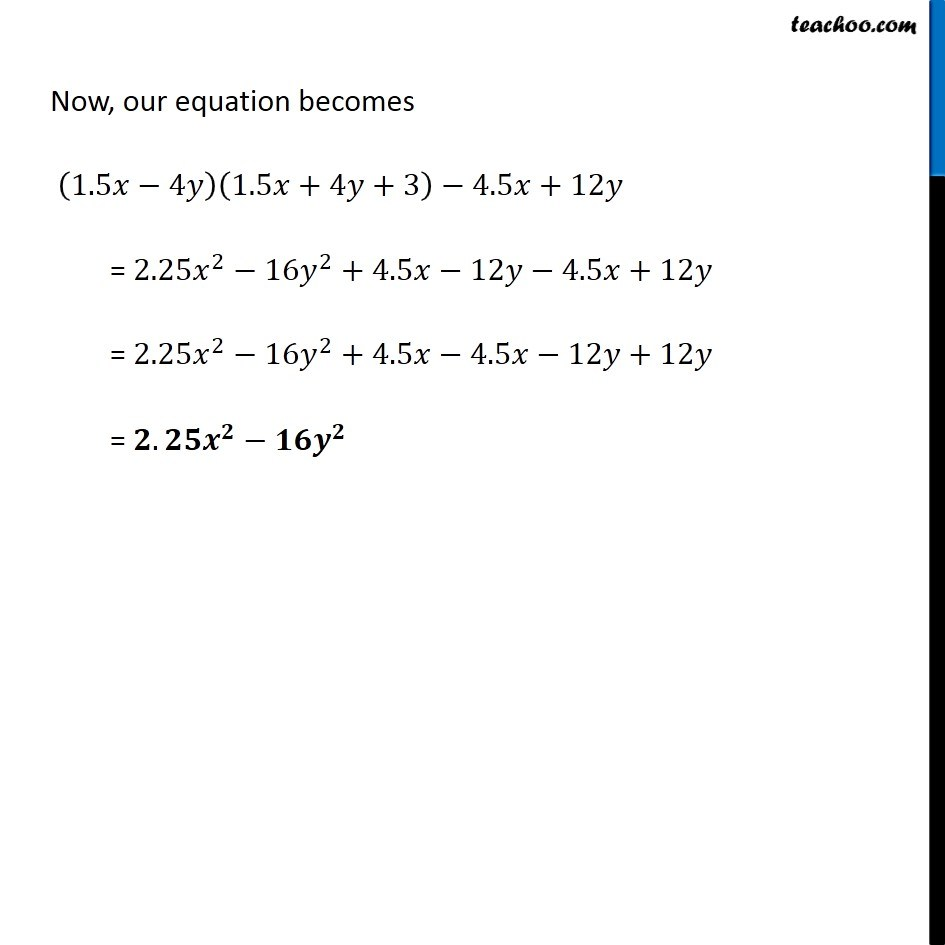Subscribe to our Youtube Channel - https://you.tube/teachoo

1. Chapter 9 Class 8 Algebraic Expressions and Identities
2. Concept wise
3. Multiplication of Polynoimals by Polynomials

Transcript

Ex 9.4, 3 Simplify. (vii) (1.5𝑥−4𝑦)(1.5𝑥+4𝑦+3)−4.5𝑥+12𝑦 Here, there are 2 expressions: First expression = (1.5𝑥−4𝑦)(1.5𝑥+4𝑦+3) Second expression = 4.5𝑥+12𝑦 Solving First expression (1.5𝑥−4𝑦)(1.5𝑥+4𝑦+3) = 1.5𝑥(1.5𝑥+4𝑦+3)−4𝑦(1.5𝑥+4𝑦+3) = (1.5𝑥×1.5𝑥)+(1.5𝑥×4𝑦)+(1.5𝑥×3)−(4𝑦×1.5𝑥) −(4𝑦×4𝑦)+(4𝑦×3) = (15/10×15/10) 𝑥^2+(15/10×4)𝑥𝑦+(15/10×3)𝑥−(4×15/10)𝑦𝑥 −16𝑦^2+12𝑦 = (225/100) 𝑥^2+(60/10)𝑥𝑦+(45/10)𝑥−(60/10)𝑦𝑥−16𝑦^2+12𝑦 = 2.25𝑥^2+6𝑥𝑦+4.5𝑥−6𝑦𝑥−16𝑦^2+12𝑦 = 2.25𝑥^2−16𝑦^2+4.5𝑥−12𝑦+6𝑥𝑦−6𝑥𝑦 = 2.25𝑥^2−16𝑦^2+4.5𝑥−12𝑦 Now, our equation becomes (1.5𝑥−4𝑦)(1.5𝑥+4𝑦+3)−4.5𝑥+12𝑦 = 2.25𝑥^2−16𝑦^2+4.5𝑥−12𝑦−4.5𝑥+12𝑦 = 2.25𝑥^2−16𝑦^2+4.5𝑥−4.5𝑥−12𝑦+12𝑦 = 𝟐.𝟐𝟓𝒙^𝟐−𝟏𝟔𝒚^𝟐

Multiplication of Polynoimals by Polynomials